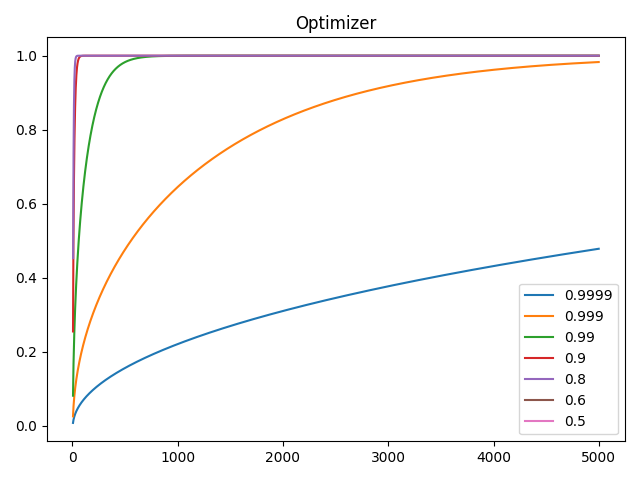This implementation is based on the official implementation of the paper On the Variance of the Adaptive Learning Rate and Beyond.

We have implemented it in PyTorch as an extension to our AMSGrad implementation thus requiring only the modifications to be implemented.

Adam optimizer sometimes converges to a bad local optima during the initial stages of the training; especially when training transformers. Researches use warmups to counter this; for the the initial training steps (warm-up stage) they use a low learning rate. This paper identifies the problem to be the high variance of adaptive learning rate during initial stages of training, and counters it using a new rectification term to reduce variance.

The paper also evaluates two variance reduction mechanisms: Adam-2k: Only compute the adaptive learning rate ( in Adam) during the first 2k steps, without changing parameters or calculating momentum (). Adam-eps: Adam with large .

Let and be the functions to calculate momentum and adaptive learning rate. For Adam, they are

### Exponential moving average as simple moving average

The distribution of exponential moving average can be approximated as a simple moving average.

Here we are taking the simple moving average of the last gradients. satisfies the following,

which gives,

### Scaled inverse chi-squared

From above we have where . Note that here is the standard deviation and different from for momentum.

Scaled inverse chi-squared is the distribution of squared inverse of mean of normal distributions. where .

### Rectification

They prove that variance of decreases with when .

Therefore the variance is minimized at maximal which is . Let the minimum variance be

In order to ensure that the adaptive learning rate has consistent variance, we rectify the variance with

### Approximating

They estimate based on first order expansion of 🤪 I didn't get how it was derived.

From distribution we have,

which gives,

### Rectification term

We have

where is for . Lt and step be , and be the rectification term at step .

This gives,

139import math
140from typing import Dict, Optional
141
142import torch
143
144from labml_nn.optimizers import WeightDecay
145from labml_nn.optimizers.amsgrad import AMSGrad

This class extends from AMSAdam optimizer defined in amsadam.py .

148class RAdam(AMSGrad):

### Initialize the optimizer

• params is the list of parameters
• lr is the learning rate
• betas is a tuple of (, )
• eps is or based on optimized_update
• weight_decay is an instance of class WeightDecay defined in __init__.py
• optimized_update is a flag whether to optimize the bias correction of the second moment by doing it after adding
• amsgrad is a flag indicating whether to use AMSGrad or fallback to plain Adam
• degenerate_to_sgd whether to use sgd when the rectification term is intractable.
• defaults is a dictionary of default for group values. This is useful when you want to extend the class RAdam .
155    def __init__(self, params, lr=1e-3, betas=(0.9, 0.999), eps=1e-8,
156                 weight_decay: WeightDecay = WeightDecay(),
157                 optimized_update: bool = True,
159                 degenerated_to_sgd=True, defaults=None):
175        self.degenerated_to_sgd = degenerated_to_sgd
176        super().__init__(params, lr, betas, eps, weight_decay, optimized_update, amsgrad, defaults)

### Take an update step for a given parameter tensor

• state is the optimizer state of the parameter (tensor)
• group stores optimizer attributes of the parameter group
• grad is the current gradient tensor for the parameter
• param is the parameter tensor
178    def step_param(self, state: Dict[str, any], group: Dict[str, any], grad: torch.Tensor, param: torch.nn.Parameter):

Calculate weight decay

189        grad = self.weight_decay(param, grad, group)

Get and ; i.e. and without bias correction

192        m, v = self.get_mv(state, group, grad)

Calculate the number of optimizer steps

195        state['step'] += 1

198        self.r_adam_update(state, group, param, m, v)

### Calculate rectification term

200    @staticmethod
201    def calc_rectification_term(beta2: float, step: int) -> Optional[float]:
207        beta2_t = beta2 ** step
209        rho_inf = 2 / (1 - beta2) - 1
211        rho = rho_inf - 2 * step * beta2_t / (1 - beta2_t)

is tractable when . We are being a little more conservative since it's an approximated value

215        if rho >= 5:
217            r2 = (rho - 4) / (rho_inf - 4) * (rho - 2) / rho * rho_inf / (rho_inf - 2)
218            return math.sqrt(r2)
219        else:
220            return None

### Do the RAdam parameter update

• state is the optimizer state of the parameter (tensor)
• group stores optimizer attributes of the parameter group
• param is the parameter tensor
• m and v are the uncorrected first and second moments and ; i.e. and without bias correction
222    def r_adam_update(self, state: Dict[str, any], group: Dict[str, any], param: torch.nn.Parameter,
223                      m: torch.Tensor, v: torch.Tensor):

Get and

235        beta1, beta2 = group['betas']

Bias correction term for ,

237        bias_correction1 = 1 - beta1 ** state['step']

Bias correction term for ,

239        bias_correction2 = 1 - beta2 ** state['step']
240
241        r = self.calc_rectification_term(beta2, state['step'])

Get learning rate

244        lr = self.get_lr(state, group)

If is intractable

247        if r is not None:

Whether to optimize the computation by combining scalar computations

249            if self.optimized_update:

Denominator

251                denominator = v.sqrt().add_(group['eps'])

Step size

253                step_size = lr * math.sqrt(bias_correction2) * r / bias_correction1

Update parameters

256                param.data.addcdiv_(m, denominator, value=-step_size)

Computation without optimization

258            else:

Denominator

260                denominator = (v.sqrt() / math.sqrt(bias_correction2)).add_(group['eps'])

Step size

262                step_size = lr * r / bias_correction1

Update parameters

265                param.data.addcdiv_(m, denominator, value=-step_size)

If is intractable do a SGD with momentum

268        elif self.degenerated_to_sgd:

Step size

270            step_size = lr / bias_correction1

Update parameters

273            param.data.add_(m, alpha=-step_size)

### Plot against for various276def _test_rectification_term():
282    import matplotlib.pyplot as plt
283    import numpy as np
284
285    beta2 = [0.9999, 0.999, 0.99, 0.9, 0.8, 0.6, 0.5]
286    plt.plot(np.arange(1, 5_000), [[RAdam.calc_rectification_term(b, i) for b in beta2] for i in range(1, 5_000)])
287    plt.legend(beta2)
288    plt.title("Optimizer")
289    plt.show()
290
291
292if __name__ == '__main__':
293    _test_rectification_term()